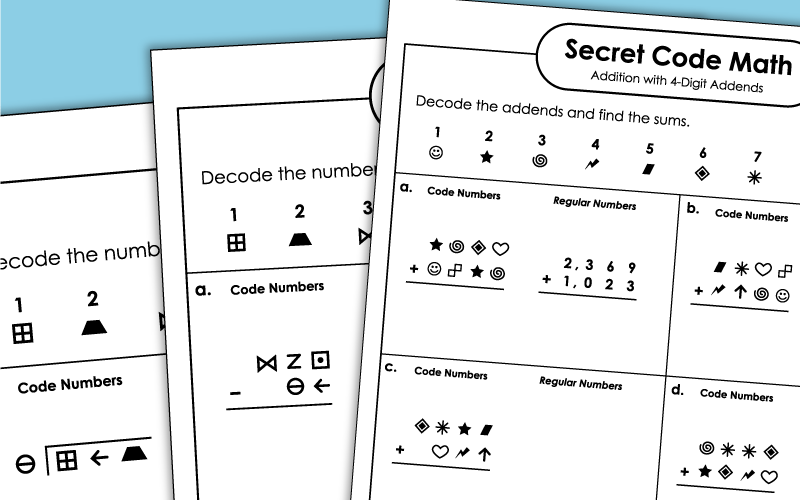# Secret Code Math

The printable "Secret Code Math" worksheets below cover dozens of different math topics, including addition, multiplication, division, and subtraction. Students first decode the numbers in the problems, then they solve.On this worksheet, students will decipher the code to create 3-digit addition problems. Then they'll solve each one.
To solve these addition problems, students will first need to decipher the symbol addends.
This column addition worksheet has three addends. Each one has 2 or 3 digits.
These coded problems have up to 4 addends, with 2, 3, or 4 digits in each.

## Subtraction

These secret code math subtraction problems each have 2-digits. Students do not borrow or regroup to solve.
Students will need to borrow/regroup to solve the subtraction problems on this sheet.
After decoding the numbers using the key, students will subtract to find the differences.
These 3-digit subtraction problems require students to borrow across zero. (example: 400-126)
Translate the picto-code numbers into "regular numbers" and then solve. All problems require students to subtract 4-digit numbers across zero. (example: 5,000-2,614)

## Rounding

Decode the numbers, then round each one to the nearest ten. (example: 264 rounds to 260)
On this one, children will figure out the numbers and then round to the nearest thousand.

## Comparing Numbers

Decode sets of symbols into numbers. Compare each group with "greater than," "less than," or "equal to."

## Multiplication

Decode the factors. Then write the product for each basic multiplication fact, up to 10x10.
First decode the numbers using the key at the top of the page. Then find the product for each pair of numbers.
(example: 54 x 6)
Find the products by multiplying 4-digit numbers by 1-digit numbers.
(example: 3,478 x 4)
Here you'll multiply pairs of double-digit numbers together.
(example: 84 x 51)
Multiply 3-digit numbers by 2-digit numbers in this cypher-code activity.

## Division

Convert the pictures into numbers, then write the quotient for each basic division fact.
For this activity, students will be solving problems that have 1-digit quotients with simple reminders.
These problems have 3-digit dividends, 2-digit quotients, and no remainders.
This version has 3-digit dividends and 2-digit quotients. Each problem has a remainder.
Math Worksheets

This page has an full index of math worksheet topics on our website.

Scoot!

Scoot! is a whole-class math game that will have students moving from desk to desk as they solve math problems.

Single & Multi-Step Word Problems

Here you'll find a collection of word problems for all grade levels.

## Worksheet PicturesMy Account
Site Information